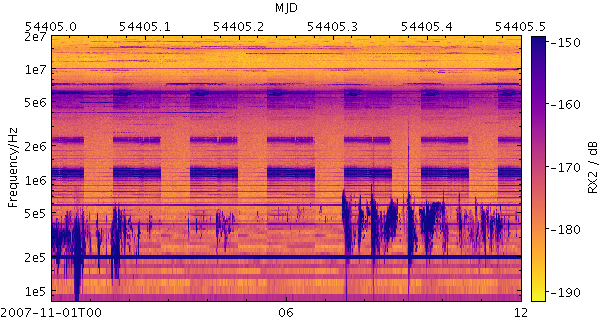Next Previous Up Contents
Up: Layer Types
Previous: yerror

#### 8.3.48 `spectrogram`

Plots spectrograms. A spectrogram is a sequence of spectra plotted as vertical 1-d images, each one plotted at a different horizontal coordinate.

This specialised layer is only available for `time` plots.

The way that data values are mapped to colours is usually controlled by options at the level of the plot itself, rather than by per-layer configuration.

Usage Overview:

```   layerN=spectrogram tN=<time-expr> ttypeN=DecYear|MJD|JD|Unix|Iso8601
spectrumN=<array-expr> twidthN=<num-expr> inN=<table>
ifmtN=<in-format> istreamN=true|false icmdN=<cmds>
```

All the parameters listed here affect only the relevant layer, identified by the suffix `N`.

Example:```   stilts plot2time layer1=spectrogram in1=LRS_NPW_V010_20071101.cdf t1=epoch spectrum1=RX2
t2func=mjd t2label=MJD
auxfunc=linear auxmap=plasma auxclip=0,1
xpix=600 ypix=320
tmin=2007-11-01T00 tmax=2007-11-01T12 ymax=480```

`icmdN = <cmds>`       (ProcessingStep[])
Specifies processing to be performed on the layer N input table as specified by parameter `inN`. The value of this parameter is one or more of the filter commands described in Section 6.1. If more than one is given, they must be separated by semicolon characters (";"). This parameter can be repeated multiple times on the same command line to build up a list of processing steps. The sequence of commands given in this way defines the processing pipeline which is performed on the table.

Commands may alteratively be supplied in an external file, by using the indirection character '@'. Thus a value of "`@filename`" causes the file `filename` to be read for a list of filter commands to execute. The commands in the file may be separated by newline characters and/or semicolons, and lines which are blank or which start with a '`#`' character are ignored.

`ifmtN = <in-format>`       (String)
Specifies the format of the input table as specified by parameter `inN`. The known formats are listed in Section 5.1.1. This flag can be used if you know what format your table is in. If it has the special value `(auto)` (the default), then an attempt will be made to detect the format of the table automatically. This cannot always be done correctly however, in which case the program will exit with an error explaining which formats were attempted. This parameter is ignored for scheme-specified tables.

[Default: `(auto)`]

`inN = <table>`       (StarTable)
The location of the input table. This may take one of the following forms:
• A filename.
• A URL.
• The special value "`-`", meaning standard input. In this case the input format must be given explicitly using the `ifmtN` parameter. Note that not all formats can be streamed in this way.
• A scheme specification of the form `:<scheme-name>:<scheme-args>`.
• A system command line with either a "`<`" character at the start, or a "`|`" character at the end ("`<syscmd`" or "`syscmd|`"). This executes the given pipeline and reads from its standard output. This will probably only work on unix-like systems.
In any case, compressed data in one of the supported compression formats (gzip, Unix compress or bzip2) will be decompressed transparently.
`istreamN = true|false`       (Boolean)
If set true, the input table specified by the `inN` parameter will be read as a stream. It is necessary to give the `ifmtN` parameter in this case. Depending on the required operations and processing mode, this may cause the read to fail (sometimes it is necessary to read the table more than once). It is not normally necessary to set this flag; in most cases the data will be streamed automatically if that is the best thing to do. However it can sometimes result in less resource usage when processing large files in certain formats (such as VOTable). This parameter is ignored for scheme-specified tables.

[Default: `false`]

`spectrumN = <array-expr>`       (String)
Provides an array of spectral samples at each data point. The value must be a numeric array (e.g. the value of an array-valued column).

The value is an array-valued algebraic expression based on column names as described in Section 10. Some of the functions in the Arrays class may be useful here.

`tN = <time-expr>`       (String)
Time coordinate.

The value is a Time value algebraic expression based on column names as described in Section 10.

`ttypeN = DecYear|MJD|JD|Unix|Iso8601`       (TimeMapper)
Selects the form in which the Time value for parameter `tN` is supplied. Options are:
• `DecYear`: Years since 0 AD
• `MJD`: Modified Julian Date
• `JD`: Julian Day
• `Unix`: Seconds since midnight 1 Jan 1970
• `Iso8601`: ISO 8601 string
If left blank, a guess will be taken depending on the data type of the value supplied for the `tN` value.
`twidthN = <num-expr>`       (String)
Range on the Time axis over which the spectrum is plotted. If no value is supplied, an attempt will be made to determine it automatically by looking at the spacing of the Time coordinates plotted in the spectrogram.

The value is a numeric algebraic expression based on column names as described in Section 10.

Next Previous Up Contents